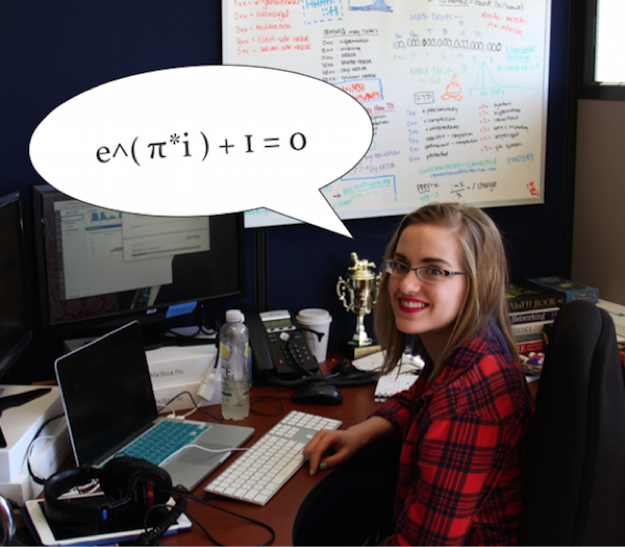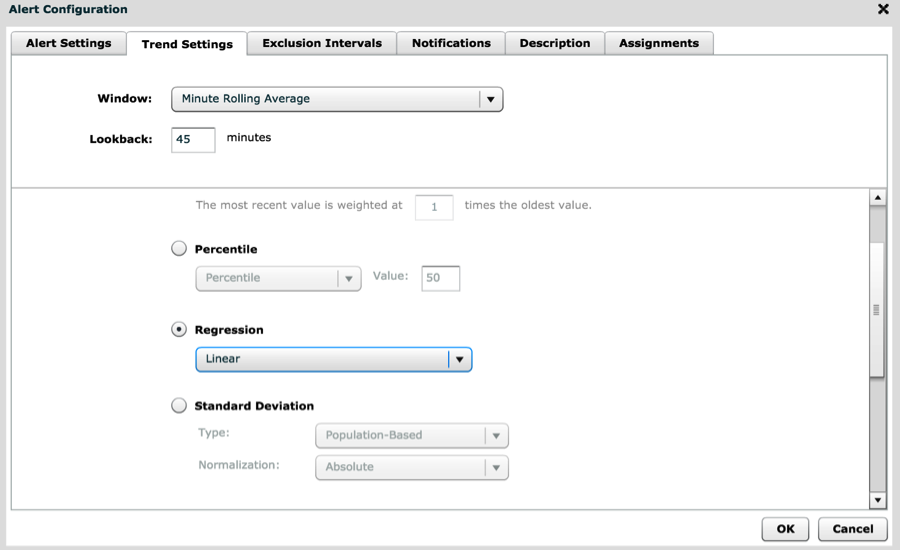###BlogAmanda Tillman is an IT Data Analyst/Support Engineer at ExtraHop

IT Operations folks have a good reason to be skeptical about alerts sent by whatever monitoring solutions they have in place on their infrastructure. If we're honest with ourselves, most alerts are pretty useless. Do you just put up with it and waste time sifting through each one, or should you turn them off altogether? If only there was a way to make our alerts smarter …

[Cue heroic trumpet blast]

There's hope! As it turns out, math can help us make our alerts smarter. The problem with most trend-based alerts is that they use a mathematically fuzzy simple mean/average that is only sufficient in some situations. The more versatile you can be in summarizing a data set, the better off you'll be in efficiently understanding overall data trends and making your alerts more useful.

Need to watch processing time for database calls? You'll want to use a linear average weighting model. Want to catch broadcast storms on the network? Use the double exponential. Interested in trending against daily/weekly traffic fluctuations? Use a regression.## Weighting Models for Various Scenarios

The weighting model options available for trend lines in the ExtraHop platform are perfect for doing just this type of alert optimization. Below, I've outlined what's going on mathematically for each weighting model available in ExtraHop, as well as some of their strengths and weaknesses and common use cases.

### Mean

The "average" that is intended to summarize the set of data points. There are a few options on how to calculate this ...

• Linear Average – Same as the arithmetic mean, i.e. adding all the values up and dividing by the count of how many metrics are in the set. Not useful in data sets with large variance, but excellent where results are tightly clustered. Processing time for database calls is one example, where most results at 0-5ms with much larger outliers (replication, data offload, bulk reporting, etc). Alerting on the mean minimizes the "less important" outlying values.
• Single Exponential – Accounts for averages over a period of time, and makes the newer averages more "significant" in the calculation of the mean. Good in cases when data is unpredictable, and you want newer trends to be prominent. The Single Exponential option helps with tactical alerting during short-term problem management. Some of the most difficult troubleshooting exercises center on degradations that occur suddenly and sporadically, so weighting recent results more heavily can account for the unusual metric fluctuations that precede such a degradation. *
• Double Exponential – Looks at previous rate changes, trends those rates, and predicts what the most likely rate change for the data set is. Good to use when you want to be warned that something is "spinning" out of control. If you were monitoring network metrics like ARP packet rate, packet count, etc., the Double Exponential option would help to detect broadcast storms or sudden saturation from competing services on the network. *
• "The most recent value is weighed at x times the oldest value" option helps you configure how powerful you want observations in the past to be when calculating the mean. (Larger values for x will make past metrics less significant.)

### Percentile

Shows where a portion (percentage) of all metrics in the data set lie.

• Percentile (value) – Answers the question, "What value are x% of all metrics below?" Percentile (value) metrics are definitely Part of Your Complete Monitoring Strategy™. In particular, tracking 85th, 90th, 95th and 99th percentile for network latency and processing time can speak volumes about the consistency of service delivery and how badly the service degrades; you'll also get a view into what proportion of the user population suffers from degraded performance. Percentile metrics surface "invisible" performance problems that can dog an application for months (if not years).
• Min Value – Minimum (least) value of metrics.
• Max Value – Maximum (greatest) value of metrics. If an application is only supported up through a certain bandwidth, you can use a max measurement to easily tell when someone exceeds that limit.

### Regression

Finds the "line of best fit" and answers the question, "Given the data I've observed, what trends exist?" The Regression weighting model is useful for trending against daily traffic fluctuations (i.e. increasing from 7am-10am, peak at 10:30/11:00, lunch break, afternoon peak, etc.) or week versus weekend traffic volumes.

• Linear – Finds the trending line (y=mx+b) that has the least amount of "error" in comparison to the actual, observed values (sum of least squares). This method results in portions of straight lines, which is good to use when you expect consistency in data (metrics that are constantly increasing/decreasing/not changing).
• 2nd Degree Polynomial – Same idea of a linear regression, but this regression measures and graphs the rate of change (trending line represents the degree to which metrics are increasing/decreasing). This results in a hyperbolic (i.e. curved) function. The 2nd Degree Polynomial option allows for more variation in trending, and can "bend"/accommodate more to whatever trend is being observed.*

### Standard Deviation

Describes how "concentrated" data is. The "type" option picks how the standard deviation is calculated. The "normalization" option allows an extra option to modify standard deviation to a standard scale. Note that technically this measurement is only "valid" on normally distributed data sets, but it can still be used anywhere to give you a general idea of what data looks like.

Standard Deviation Type Options:

• Population Based – Use if you "have" all the data you want to analyze in the trend, or if the data you're feeding to the ExtraHop is all you care about.
• Sample Based – Makes an estimate about everything else going on based on the data you're looking at. Utilize this option if you want to analyze your data as a portion of the environment, and use the results to make a statement regarding the "bigger picture."

Normalization Options:

• Absolute – Graphs the standard deviation as calculated with relevant units.
• Relative to Mean – Also known as variation coefficient, this is calculated as the standard deviation divided by the mean. This measurement equates to the "number" of standard deviations away from the mean you are, whereas "absolute" is a measurement of the actual standard deviation relative to the mean. "Relative to mean" is a good option if you want to compare standard deviations across different environments, where the averages/means may not compare nicely.

### Static Value

Super simple. this one just graphs a straight line at the specified value. Good for SLAs or any performance against a goal. Most executive reports should include a static value of some sort.

### Time Delta

This option is not necessarily mathematically challenging, but can still provide valuable insight. If you want to compare current metrics to those seen in a previous time window, use this option. The comparison window is based off of the time window you have set on the main "trend line" tab. So, for example, if you trend is for HTTP requests "same hour of day, 10 day look back," then the trend value is the difference between the current HTTP request volume and the volume of HTTP requests collected during that same hour of the day exactly 10 days ago.

### Trimean

Find the median (value at 50% of all metrics, known as Q2) and two quartiles (values at 25% and 75% of all metrics, known as Q1 and Q3 respectively). Trimean = (Q1 + (2*Q2) + Q3) / 4. [Trivia: Trimean is better than quadmean, quintmean, etc. because three measurement points are the "most efficient" at measuring without having diminishing returns with regards to accuracy. The more you know!]

### Winsorized Mean

Useful when you want to eliminate the effect of outliers. Depending on the threshold, you replace the highest and lowest values of the dataset with their closest "neighbors" and then calculate the mean on the new data set. This weighting model is useful for ignoring the impact of health checks (which tend to be artificially fast) or end users with terrible network connections (which tend to artificially skew averages northward).

Threshold options for the Winsorized Mean are as follows:

• 10th/90th percentile – Replace each data point in the lowest 10% of metrics with the value of the data point just above the 10th percentile, and replace each data point in the highest 10% of metrics with the value of the data point just below the 90th percentile.
• 5th/95th percentile – Replace each data point in the lowest 5% of metrics with the value of the data point just above the 5th percentile, and replace each data point in the highest 5% of metrics with the value of the data point just below the 95th percentile.
• 25th/75th percentile – Replace each data point in the lowest 25% of metrics with the value of the data point just above the 25th percentile, and replace each data point in the highest 25% of metrics with the value of the data point just below the 75th percentile.
\* Note – Any type of exponential (2nd degree) trending option will require three data points to graph. Math-y explanation: A trend is composed of two points. So in order to compare trends and graph them, you'll need at least two trends to look at (kind of like how you need at least two points to make a line). Put two trends back to back, and you'll have a minimum of three points since you can use the center point as the end of one trend, and the beginning of the next.